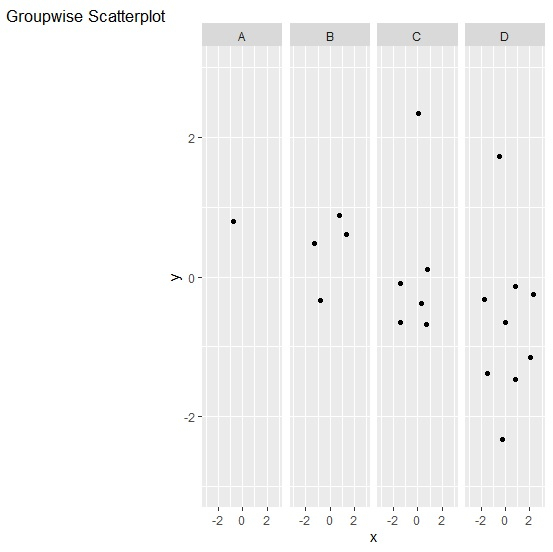# How to write plot description outside plot in facetted plot using ggplot2 in R?

To write plot description outside plot using ggplot2, we can use annotate function and coord_cartesian function. The annotate function will define the plot description and the coord_cartesian function will define the position of the text outside the plot area.

Check out the below given example to understand how it works.

## Example

Following snippet creates a sample data frame −

x<-rnorm(20)
y<-rnorm(20)
grp<-sample(LETTERS[1:4],20,replace=TRUE)
df<-data.frame(x,y,grp)
df

The following dataframe is created −

      x            y         grp
1   0.78077456  -1.46198018  D
2  -0.49452689   1.72471204  D
3  -1.39619653  -0.64859852  C
4  -0.73260404   0.79430612  A
5   0.83483420  -0.12772415  D
6  -1.42229860  -0.09636467  C
7   0.02591011   2.35094791  C
8   0.02007910  -0.64553958  D
9   0.78893141   0.89014539  B
10  0.28600357  -0.37031710  C
11 -1.69337019  -0.31425710  D
12  2.08396139  -1.14526981  D
13  0.74312431  -0.68121481  C
14  1.33009148   0.61906318  B
15  0.75865211   0.10783624  C
16 -0.82221628  -0.32764999  B
17 -0.28765766  -2.32935412  D
18 -1.30783428   0.48349510  B
19  2.32201111  -0.25456548  D
20 -1.50377138  -1.37306174  D

To load ggplot2 package and create facetted scatterplot between x and y with plot description outside the plot area, add the following code to the above snippet −

library(ggplot2) ggplot(df,aes(x,y))+geom_point()+facet_grid(.~grp)+labs(tag="Groupwise Scatterplot")+coord_cartesian(xlim=c(-3,3),ylim=c(-3,3),clip = "off")

## Output

If you execute all the above given snippets as a single program, it generates the following output −Updated on: 22-Nov-2021

270 Views